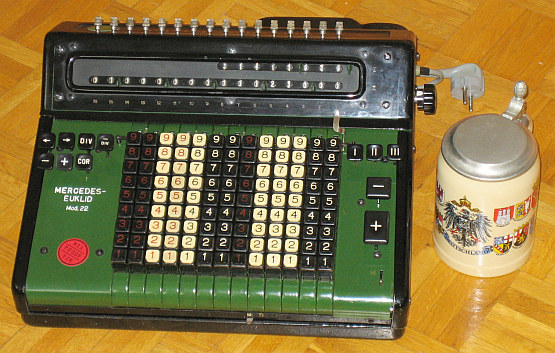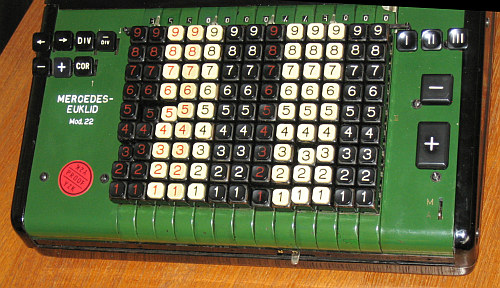previous <<==>> next

MERCEDES-EUKLID 22In 2006 the EUKLID-22 came as "Motor is humming - but nothing works..."
Restoration is not only a matter of skills - also of patience:
Have a special look at the (missing) left [+] key: Hand-Made !

Have a look at the Restoration Procedure...

(*) Spring 2017 = New Picture:
Collector-Friend G.B. asked me to correct my wrong KEY-SEQUENCE ...
Right Sequence:   Br-Br-Wr-Wr-Wb-Bw-Bw-Br-Wb-Wb-Wb-Bw-Bw
( B;b = black / W;w = white / r = red )
 ``` TECHNICAL DATA of the Electro-Mechanical Calculator MERCEDES-EUKLID *************************************************************************** Invented by CHRISTEL HAMANN in 1906 Model » 22 « Serial-Number: 24943 Dimensions: (ca.) Width = 14_1/2" / 37 cm Depth = 14_1/2" / 37 cm Height = 6_3/4" / 17 cm Weight: (ca.) 34 lbs / 15.5 kg Power Supply: 220 V / 100 W (grounded, AC ) Mechanics: 13*9 Full-Keyboard Proportional-Lever / Proportional-Hebel Functions: Add, Subtract, Multiply, Divide Division-Automatic! Registers: I Counter = 8 Decimals II Arithmetic = 16 Decimals III Input = 13 Decimals Manufacturer: MERCEDES BUEROMASCHINEN WERKE Zella-Mehlis in Thueringen Germany 1933 BASICS & HOW TO USE the MERCEDES-EUKLID 22 ********************************************** (A) Clearing Registers: ----------------------- The upper right keys [I],[II],[III] clear registers: (I)&(II) by motor power; key [III] clears the keyboard manually. There is a lever [N/D] below the keyboard ( N=Normal; D=Division ): When set to "N" and the lever [M/A] on the right side is set to "A", than ALL KEYS will be CLEARED when pressed [+] or [III] Key. In right position "D" the clearing is incomplete: Only 6 columns on the right side keep their key positions - SEE EXAMPLE(*)DOWN ... The right [M/A] repeat-lever (up="M"=Multiplication, down="A"=Addition) works with the right [+] & [-] keys: In its (A) position the input (III) register will be cleared after each (+) or (-) operation, but in (M) position the input is hold - The [+] or [-] keys on the left side don't clear the input, undependent of the repeat lever position. (B) Shifting the Carriage: -------------------------- The upper left keys [<-] or [->] shift the carriage by motor power to the left or to the right. (C) Carriage Settings & Counting Directions: -------------------------------------------- The arithmetic register (II) is PRE-SETable with 16 metal knobs on top of the calculator. The [COR] key, left beside the keyboard, controls the counter (I) and has 3 positions: When not pressed (= DEFAULT), register (I) counts "positive"; when pressed in full (I) counts "negative" and in half-position register (I) doesn't count at all. "Positive" means the counter & arithmetic unit are working in the same; in case of "negative", both are working in opposite direction. To release the key, push it horizontally. Prior to each task the registers (I), (II), (III) have to be cleared; Default settings: Carriage in the leftmost position, repeat-lever down. ADDITION & SUBTRACTION: ======================= Example: 123 + 45 - 6 = 162 ADD: Enter the first number (123) in the far right columns. Press the right [+] key to transfer the number into arithmetic unit. The counting unit displays the figure 1. Enter the second number (45). Press the right [+] key to add the number. The arithmetic unit displays the intermediate sum (168) and the counting unit displays the figure 2. SUBTRACT: Enter the third number (6). Press the right [-] key. The arithmetic unit displays the result (162) and the counting unit is decreased by 1. REMARK: NEGATIVE RESULTS are displayed in the arithmetic unit as the COMPLEMENT of the next higher 10, 100, 1000, ... Example: -12 = 99...9988 MULTIPLICATION: =============== Example: 123 x 45 = 5535 If the right [+] or [-] keys will be used, set the repeat-lever to "M". Enter the multiplicand (123) in the far right columns. The multiplicator (45) has two digits, so the arithmetic unit is moved to position 2 with the [->] key. Repeat pressing the [+] key, until the first figure of the multiplicator (4) will appear in the 2nd position of the counter unit. Move the arithmetic unit with the [<-] key to pos. 1. Repeat pressing the [+] key, until the second figure of the multiplicator (5) appears in the 1st position of the counter unit. The multiplication is done: The multiplicand (123) stays in keyboard, the multiplicator (45) is in the counter unit and the result (5535) is in the arithmetic unit. DIVISION: ========= Example: 22 : 7 = 3.1428571 Remainder 3 Division requires 3 steps: (A) To Set the Dividend into Arithmetic Unit: ----------------------------------------- Clear registers [I], [II], [III]. For the maximum number of decimals, shift the carriage to the far right position, using [>] key. PRE-SET the dividend (22) in columns 9 & 8 of the arithmetic unit with the KNOBS on TOP. (B) To Set the Divisor in the Keyboard: ----------------------------------- Enter the divisor (7) right ( columne 1 ) of the input unit, below the dividend (22). (C) To Divide: ---------- Press the (upper, left) Divide [DIV] key and the machine will calculate all decimals (and moves the carriage) automatically. The result (3.1428571) is in the counter (I) unit, and the remainder (3) is in the arithmetic (II) unit. The divisor (7) stays in the keyboard, therefore an additional decimal can be estimated... ( 4, cause 4 x 7 = 28 ). Have a look at "Calculating Trickies" ... R E M A R K S : =============== (a) Pressing the [DIV] key shift the carriage automaticaly to the far-right position at first - BUT: To make sure, that divident and divisor are right aligned, this feature is not used here! (b) In case of a looping machine (e.g. dividing through zero), "touch" the [/DIV] key to release the [DIV] key ... (c) The [/DIV] key calculates the 9-COMPLEMENT. (*) Collector-Friend G.B. asked me to correct my wrong KEY-SEQUENCE ... -------------------------------------------------------------------------- On BBST-Meeting, 6th May 2017, Gerwin Bertelmann gave the T A L K : »LEVER N-D OF THE MERCEDES-EUKLID 21/22 + BLACK-RED & WHITE-RED KEYS« In front of the Keyboard is the LEVER [N/D] ( N=Normal; D=Division ). When set to "N" and the LEVER [M/A] on the right side is set to "A", than ALL KEYS will be DELETED when pressed [+] or [III] Key. In Position "D" only the "LEFT HALF" of the KEYBOARD is CLEARED, ( means "LEFT-OF-THE BLACK-RED COLUMNE" ) - The right half stays !!! APPLICATION = DIVISION: SET LEVER [N/D] to "D" & Lever [M/A] to "A" ======================================================================= Example: 355/113 = 3.1415929Set DIVIDENT in LEFT SIDE & Set DIVISOR in RIGHT SIDE of the KEYBOARD. PRESS [+] Key: Divident & Divisor will be in the Arithmetic (II) Unit, Counter (I) Unit is set to "1" and Divident-KEYS (= left) are DELETED, Divisor-KEYS (= right) STAY. PRESS [-] Key: Divisor will be deleted in Arithmetic (II) Unit; the "1" will be deleted in the Counter (I) Unit. PRESS [DIV] KEY to activate AUTOMATIC DIVISION ... 355/113 = 3.1415929 "THANK YOU! G.B." impressum: *************************************************************************** © C.HAMANN http://public.BHT-Berlin.de/hamann 06/08/17 ```